Capacitor

What is capacitor and capacitor calculations.

What is capacitor

Capacitor is an electronic component that stores electric charge. The capacitor is made of 2 close conductors (usually plates) that are separated by a dielectric material. The plates accumulate electric charge when connected to power source. One plate accumulates positive charge and the other plate accumulates negative charge.

The capacitance is the amount of electric charge that is stored in the capacitor at voltage of 1 Volt.

The capacitance is measured in units of Farad (F).

The capacitor disconnects current in direct current (DC) circuits and short circuit in alternating current (AC) circuits.

Capacitor pictures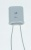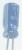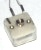Capacitor symbols

CapacitorPolarized capacitor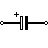Variable capacitor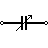Capacitance

The capacitance (C) of the capacitor is equal to the electric charge (Q) divided by the voltage (V):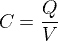C is the capacitance in farad (F)

Q is the electric charge  in coulombs (C), that is stored on the capacitor

V is the voltage between the capacitor's plates in volts (V)

Capacitance of plates capacitor

The capacitance (C) of the plates capacitor is equal to the permittivity (ε) times the plate area (A) divided by the gap or distance between the plates (d):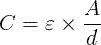C is the capacitance of the capacitor, in farad (F).

ε is the permittivity of the capacitor's dialectic material, in farad per meter (F/m).

A is the area of the capacitor's plate in square meters (m2].

d is the distance between the capacitor's plates, in meters (m).

Capacitors in series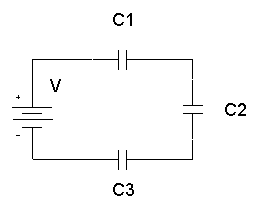The total capacitance of capacitors in series, C1,C2,C3,.. :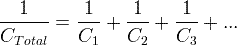Capacitors in parallel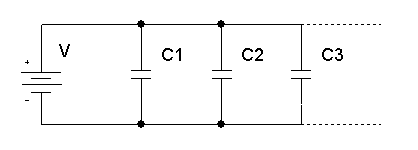The total capacitance of capacitors in parallel, C1,C2,C3,.. :

CTotal = C1+C2+C3+...

Capacitor's current

The capacitor's momentary current ic(t) is equal to the capacitance of the capacitor,

times the derivative of the momentary capacitor's voltage vc(t):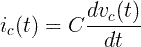Capacitor's voltage

The capacitor's momentary voltage vc(t) is equal to the initial voltage of the capacitor,

plus 1/C times the integral of the momentary capacitor's current ic(t) over time t: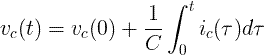Energy of capacitor

The capacitor's stored energy EC in joules (J) is equal to the capacitance C in farad (F)

times the square capacitor's voltage VC in volts (V) divided by 2:

EC = C × VC 2 / 2

AC circuits

Angular frequency

ω = 2π f

f  - frequency measured in hertz (Hz).

Capacitor's reactance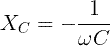Capacitor's impedance

Cartesian form: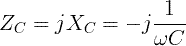Polar form:

ZC = XC∟-90º

Capacitor types

 Variable capacitor Variable capacitor has changeable capacitance Electrolytic capacitor Electrolytic capacitors are used when high capacitance is needed. Most of the electrolytic capacitors are polarized Spherical capacitor Spherical capacitor has a sphere shape Power capacitor Power capacitors are used in high voltage power systems. Ceramic capacitor Ceramic capacitor has ceramic dielectric material. Has high voltage functionality. Tantalum capacitor Tantalum oxide dielectric material. Has high capacitance Mica capacitor High accuracy capacitors Paper capacitor Paper dielectric material

Currently, we have around 1976 calculators, conversion tables and usefull online tools and software features for students, teaching and teachers, designers and simply for everyone.